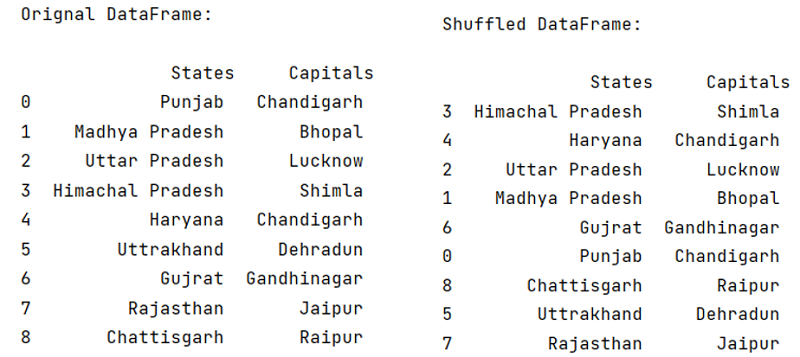# Pandas – How to shuffle a DataFrame rows?

Given a DataFrame, we have to shuffle its rows.
Submitted by Pranit Sharma, on April 13, 2022

Shuffling of rows means changing the sequence of rows randomly. Pandas allow us to shuffle the order or rows using the sample() method. We will be using the sample() method to randomly shuffle the order of rows in pandas DataFrame.

## pandas.DataFrame.sample() Method

The sample() method is an inbuilt method for shuffling sequences in python. Hence, in order to shuffle the rows in DataFrame, we will use DataFrame.sample() method. Shuffle method takes a sequence (list) as an input and it reorganize the order of that particular sequence.

Syntax:

```DataFrame.sample(
n=None,
frac=None,
replace=False,
weights=None,
random_state=None,
axis=None,
ignore_index=False
)

# or
DataFrame.sample(n=none, frac=none, axis= none)
```

Parameters:

• n: n is total number of rows to be shuffled.
• frac: frac is fraction of total instances needs to be returned, i.e., number of times shuffling needs to be done.
• axis: '0' or 'row' for rows and '1' or 'column' for columns.

Note: The sample() method does not work with immutable types of data types like strings.

To work with Python Pandas, we need to import the pandas library. Below is the syntax,

```import pandas as pd
```

Example:

```# Importing pandas package
import pandas as pd

# Creating a dictionary
d = {
'Haryana','Uttrakhand','Gujrat','Rajasthan','Chattisgarh'],
'Capitals':['Chandigarh','Bhopal','Lucknow','Shimla','Chandigarh',
}

# Creating a DataFrame
df = pd.DataFrame(d)

# Printing original DataFrame
print("\nOrignal DataFrame:\n\n",df)

# Shuffling the order of rows
# using the sample() method
df = df.sample(n=9)

# Printing the shuffled DataFrame
print("\nShuffled DataFrame:\n\n",df)
```

Output:

```
Orignal DataFrame:

States     Capitals
0            Punjab   Chandigarh
4           Haryana   Chandigarh
6            Gujrat  Gandhinagar
7         Rajasthan       Jaipur
8       Chattisgarh       Raipur

Shuffled DataFrame:

States     Capitals
6            Gujrat  Gandhinagar
4           Haryana   Chandigarh
7         Rajasthan       Jaipur
0            Punjab   Chandigarh
8       Chattisgarh       Raipur
```What's New (MCQs)

Top Interview Coding Problems/Challenges!

IncludeHelp's Blogs

Languages: » C » C++ » C++ STL » Java » Data Structure » C#.Net » Android » Kotlin » SQL
Web Technologies: » PHP » Python » JavaScript » CSS » Ajax » Node.js » Web programming/HTML
Solved programs: » C » C++ » DS » Java » C#
Aptitude que. & ans.: » C » C++ » Java » DBMS
Interview que. & ans.: » C » Embedded C » Java » SEO » HR
CS Subjects: » CS Basics » O.S. » Networks » DBMS » Embedded Systems » Cloud Computing
» Machine learning » CS Organizations » Linux » DOS
More: » Articles » Puzzles » News/Updates